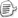### 分析表达式的逻辑

 class Program { static void Main(string[] args) { Expression> myExp = (a, b, m, n) => m * a * a + n * b * b; var calc = new BinaryExpressionCalculator(myExp); Console.WriteLine(calc.Calculate(1, 2, 3, 4)); } } class BinaryExpressionCalculator { Dictionary m_argDict; LambdaExpression m_exp; public BinaryExpressionCalculator(LambdaExpression exp) { m_exp = exp; } public double Calculate(params double[] args) { //从ExpressionExpression中提取参数，和传输的实参对应起来。 //生成的字典可以方便我们在后面查询参数的值 m_argDict = new Dictionary(); for (int i = 0; i < m_exp.Parameters.Count; i++) { //就不检查数目和类型了，大家理解哈 m_argDict[m_exp.Parameters[i]] = args[i]; } //提取树根 Expression rootExp = m_exp.Body as Expression; //计算！ return InternalCalc(rootExp); } double InternalCalc(Expression exp) { ConstantExpression cexp = exp as ConstantExpression; if (cexp != null) return (double)cexp.Value; ParameterExpression pexp = exp as ParameterExpression; if (pexp != null) { return m_argDict[pexp]; } BinaryExpression bexp = exp as BinaryExpression; if (bexp == null) throw new ArgumentException("不支持表达式的类型", "exp"); switch (bexp.NodeType) { case ExpressionType.Add: return InternalCalc(bexp.Left) + InternalCalc(bexp.Right); case ExpressionType.Divide: return InternalCalc(bexp.Left) / InternalCalc(bexp.Right); case ExpressionType.Multiply: return InternalCalc(bexp.Left) * InternalCalc(bexp.Right); case ExpressionType.Subtract: return InternalCalc(bexp.Left) - InternalCalc(bexp.Right); default: throw new ArgumentException("不支持表达式的类型", "exp"); } } }

 string InternalPrefix(Expression exp) { ConstantExpression cexp = exp as ConstantExpression; if (cexp != null) return cexp.Value.ToString(); ParameterExpression pexp = exp as ParameterExpression; if (pexp != null) return pexp.Name; BinaryExpression bexp = exp as BinaryExpression; if (bexp == null) throw new ArgumentException("不支持表达式的类型", "exp"); switch (bexp.NodeType) { case ExpressionType.Add: return "+ " + InternalPrefix(bexp.Left) + " " + InternalPrefix(bexp.Right); case ExpressionType.Divide: return "- " + InternalPrefix(bexp.Left) + " " + InternalPrefix(bexp.Right); case ExpressionType.Multiply: return "* " + InternalPrefix(bexp.Left) + " " + InternalPrefix(bexp.Right); case ExpressionType.Subtract: return "/ " + InternalPrefix(bexp.Left) + " " + InternalPrefix(bexp.Right); default: throw new ArgumentException("不支持表达式的类型", "exp"); } }

 IQueryable source = ... var query = from d in source where d > 0 select d * 2;

 var query = source.Where(d => d > 0).Select(d => d * 2);

### 序列化和传输表达式

1. 生成表达式的程序与解析表达式的程序不在同一进程内（例如在客户端生成表达式，在服务端解析）。

2. 需要储存或缓存表达式，为以后多次使用做准备。

3. 需要用其他非.NET技术处理或生成表达式树。

.NET 3.5版本的表达式树本身并没有特别优雅的序列化方式，它并不支持DataContract序列化。因为表达式的种类繁多，许多表达式都和CLR具体类型相关。通常的做法是，根据自己要使用的表达式子集手动编写序列化的代码。ToString()也可以得到一个表示表达式的字符串，可惜他不是那么容易变回表达式树。所以ToString()通常仅仅作为显示和参考。

MSDN Code Gallery中有一个LuckH提供的通用Expression Tree序列化例子。它可以提供比较清晰地XML序列化结果。

### 习题

1. 基于本文提供的四则运算例子，给他加上支持一元正负运算符和函数调用的功能。这样你就能计算Math.Sin(a) + b这样的表达式了。可以先仅支持静态函数。

2. 改写这个程序，使之能根据四则运算表达式树生成javascript代码。

3. 你可以试着将以上代码扩展成一个小小的类库，能用javascript来执行你提供的四则运算表达式。

（待续）posted on 2009-08-31 11:59  装配脑袋  阅读(16753)  评论(26编辑  收藏  举报